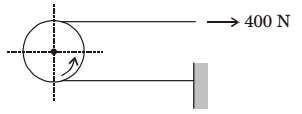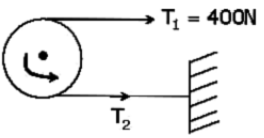## Machine Design Miscellaneous

#### Machine Design

1. A force of 400 N is applied to the brake drum of 0.5 m diameter in a band brake system as shown in figure, where the wrapping angle is 180°. If the coefficient of friction between the drum and the band is 0.25, the braking torque applied, in Nm is1.As the drum is rotating in anti clock wise direction, T1 will be right side & T2 will be clock side.

 T1 = eμθ = e0.25 × π = 2.19 T2

⇒  T2 = = 182.375 N
Braking torque = (T1 – T2 )r
= 54.4 N – m

##### Correct Option: BAs the drum is rotating in anti clock wise direction, T1 will be right side & T2 will be clock side.

 T1 = eμθ = e0.25 × π = 2.19 T2

⇒  T2 = = 182.375 N
Braking torque = (T1 – T2 )r
= 54.4 N – m

1. A band brake having bandwidth of 80 mm, drum diameter of 250 mm, coefficient of friction of 0.25 and angle of wrap of 270 degrees is required to exert a friction torque of 1000 N m. The maximum tension (in kN) developed in the band is

1. Given: b = 80 mm = 0.08 m D = 250 mm = 0.25

 R = D = 0.25 2

μ = 0.25
 θ = 270° = 270 × π = 4.712 radians 180

Ft = 100 N-m
 As p1 = eμθ p2

⇒  p1 = eμθ ⋅ p2 = e0.25 × 4.712 ⋅ p2 = 3.25p2
Now, Ft = (p1 − p2)R
 ∴  1000 =p1 − p10.125 3.25

Solving, we get maximum tension in the belt,
p1 = 11.56 kN

##### Correct Option: D

Given: b = 80 mm = 0.08 m D = 250 mm = 0.25

 R = D = 0.25 2

μ = 0.25
 θ = 270° = 270 × π = 4.712 radians 180

Ft = 100 N-m
 As p1 = eμθ p2

⇒  p1 = eμθ ⋅ p2 = e0.25 × 4.712 ⋅ p2 = 3.25p2
Now, Ft = (p1 − p2)R
 ∴  1000 =p1 − p10.125 3.25

Solving, we get maximum tension in the belt,
p1 = 11.56 kN

1. In spur gears, the circle on which the involute is generated is called the

1. NA

##### Correct Option: C

NA

1. Interference in a pair of gears is avoided, if the addendum circles of both the gears intersect common tangent to the base circles with in the points of tangency.

1. NA

##### Correct Option: A

NA

1. For a pinion of 15 teeth, under cutting ______ (increases/decreases) with _______(increase/ decrease) of pressure angle.

1. Interference decreases with increase in pressure

##### Correct Option: A

Interference decreases with increase in pressure